Lecturers Scientific Programme Participants Conference Timetable Key Dates Registration Fees Committees Social Programme Accomodation Portugal | Lisbon | Coimbra Travel Useful Links Previous Workshops Poster Book of Abstracts

Invited Speakers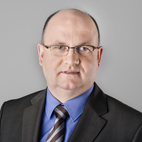Peter BennerMax Plank Institute for Dynamics ofComplex Technical SystemsGermany
 Research interests: Numerical linear algebra; Model reduction and system approximation; Parallel algorithms; Nonlinear equations, in particular algebraic Riccati equations; Linear-quadratic optimization; Robust stabilization of linear and nonlinear systems; Control of instationary PDEs; and Mathematical software.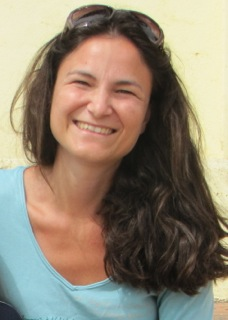Marija DodigUniversity of LisbonFaculty of ScienceLisboa, Portugal email

Research interests:   Matrix Theory and Control Theory of Linear Systems. In particular, matrix pencil completion problems; perturbation and rank distance problems; studying controllability and stability of linear systems. pplications in Engineering - signal processing, computer vision.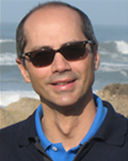Froilán M. DopicoDepartamento de MatemáticasUniversidad Carlos III de MadridSpain
 Research interests: Numerical linear algebra and Matrix theory. In particular, the development and analysis of accurate and efficient algorithms for structured matrices, matrix perturbation theory related to these algorithms and other structured problems, matrix polynomials and their linearizations, and theory and algorithms for matrix equations.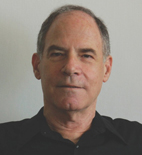Moshe GoldbergDepartment of MathematicsTechnion - Israel Institute of TechnologyIsraelemail
 Research interests: Matrix theory; Linear and multilinear algebra; Power-associative algebras; Norm theory; Numerical ranges and numerical radii; Numerical analysis of time-dependent partial diferential equations; General inequalities; Functional equations.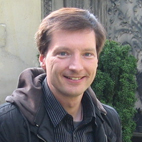Christian MehlInstitute of MathematicsTechnical University of BerlinGermany
 Research interests: Numerical and applied linear algebra, in particular: Numerical and algebraic methods for the solution of structured engenvalue problems; Quadratic engenvalue problems; Nonlinear eigenvalue problems (nonlinear in the eigenvalue parameter); Computation of canonical forms for structured matrices, matrix pencils, and matrix polynomials; Indefinite inner products and Perturbation theory.

Dietrich von Rosen (Sweden)
Title: The likelihood ratio test in bilinear models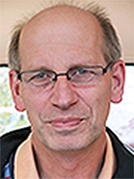Dietrich von RosenDepartment of Energy and TechnologySwedish University of Agricultural SciencesSweden
 Research interests: Inference in multivariate linear models.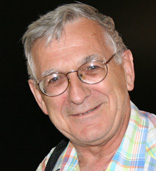Roman ZmyślonyFaculty of Mathematics, Computer Science and EconometricsUniversity of Zielona GóraPoland
 Research interests: Estimation and testing hypothesis in mixed linear models; Experimental designs; Application of experimental designs in chemistry, biology and economy.

Karol Życzkowski (Poland)
Title: Joint numerical range and numerical shadow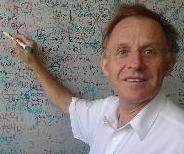Karol ŻyczkowskiAtomic Physics DepartmentInstitute of PhysicsJagiellonian UniversityPoland
 Research interests: Quantum information; Quantum measurement and entropy; Quantum chaos; Entanglement and geometry of quantum states; Random matrices; Complex Hadamard matrices; Numerical range and numerical shadow; Nonlinear dynamics and fractals; Stochastic versus chaotic dynamics; Game theory and voting power; Financial mathematics.

Young Scientists Awards - Mat Triad`2013

Jaroslav Horáček (Czech Republic)
Title: Computational complexity and interval Linear Algebra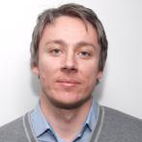Jaroslav HoráčekDepartment of Applied MathematicsFaculty of Mathematics and PhysicsCharles University in PragueCzech Republicemailwebpage
 Research interests: Interval analysis; Uncertainty; Artificial intelligence and History of mathematics.

Maja Nedović (Serbia)
Title: H-matrix theory and applications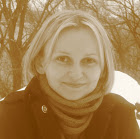Maja NedovićFaculty of Technical SciencesUniversity of Novi SadSerbia
 Research interests: Applied linear algebra.